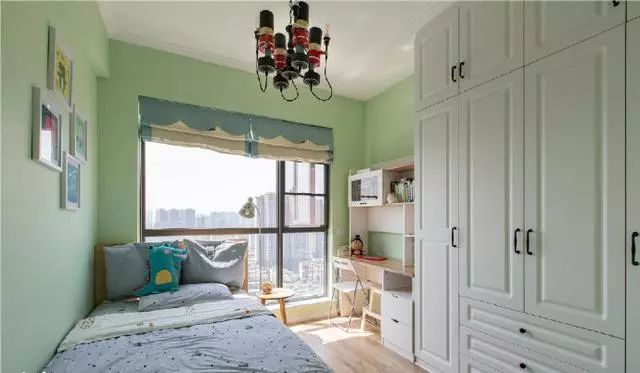# 75平米两居室，全屋通透敞亮又温馨，装修这么美，谁还愿意出门'data-lazy='1'data-height='855'data-width='640'width='640'height='auto''data-lazy='1'data-height='855'data-width='640'width='640'height='auto''data-lazy='1'data-height='690'data-width='545'width='545'height='auto''data-lazy='1'data-height='373'data-width='640'width='640'height='auto'' data-lazy='1' data-height='373' data-width='640' width='640' height='auto'' data-lazy='1' data-height='356' data-width='640' width='640' height='auto'' data-lazy='1' data-height='373' data-width='640' width='640' height='auto'' data-lazy='1' data-height='363' data-width='640' width='640' height='auto'' data-lazy='1' data-height='361' data-width='640' width='640' height='auto'' data-lazy='1' data-height='859' data-width='640' width='640' height='auto'

75平米两居室，全屋通透敞亮又温馨，装修这么美，谁还愿意出门！不知道大家喜欢这套小户型装修吗？说实话，我觉得咱们老百姓过日子，房屋装修最看重的就是要实用，然后要有家的感觉，这套房子刚好都符合，希望给大家带来一些参考价值。

-